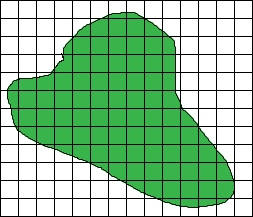SEARCH HOMEMath Central Quandaries & QueriesQuestion from Tom: I manage a golf course, and we are about to over seed the course for the upcoming winter months. We will be over seeding all greens, fairways, and tee boxes. None of the area that we will be placing seed on is a regular shape. The closest shapes they would be is a circle, rectangle or square, but most of them are all odd CURVED shapes. I know what the equation is for Square ft., and I know how many Square ft. are in an acre, but I need to be precise with the amount of seed I order. Is there a way I can calculate correct square footage for odd shapes.Tom,

If you have an accurate scale drawing of the course you should be able to use it to calculate a good approximation of the area. You could overlay a grid on the drawing, count the number of squares in the grid that are inside the regions to be seeded, use the number of squares to calculate the area of the drawing that represents the region to be seeded and then use the drawing scale to convert this area to the square footage on the course.There is a device called a planimeter that you could use with a drawing also.

HarleyMath Central is supported by the University of Regina and The Pacific Institute for the Mathematical Sciences.